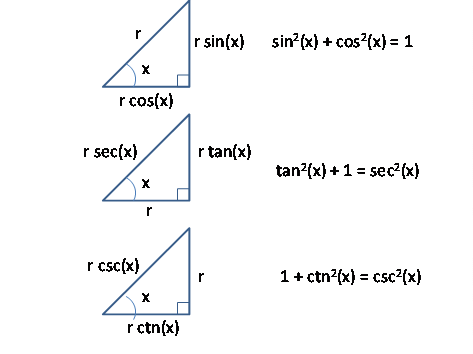Trigonometric Functions

INTRODUCTION

Trigonometric functions are defined on an arbitrary right triangle shown below:TRIGONOMETRIC FUNCTIONS

The trigonometric functions are all six ratios of the lengths of the sides of a right triangle.  These ratios are a function only of the angle “x” meaning that they are independent of the actual size of the triangle.  These must be FIRMLY committed to memory.   Note that in practice, the first three functions,  {sine, cosine, and tangent}, are by far the most important.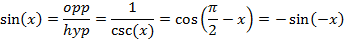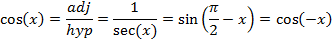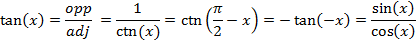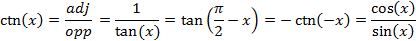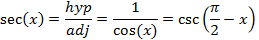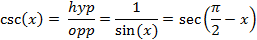PYTHAGOREAN THEOREM

In practice, these relations for the sides of a triangle are most often used in science and engineering.  Basically they express the length of the sides of a right triangle in trigonometric functions when the absolute length of one side is known.  It is essential to memorize them.